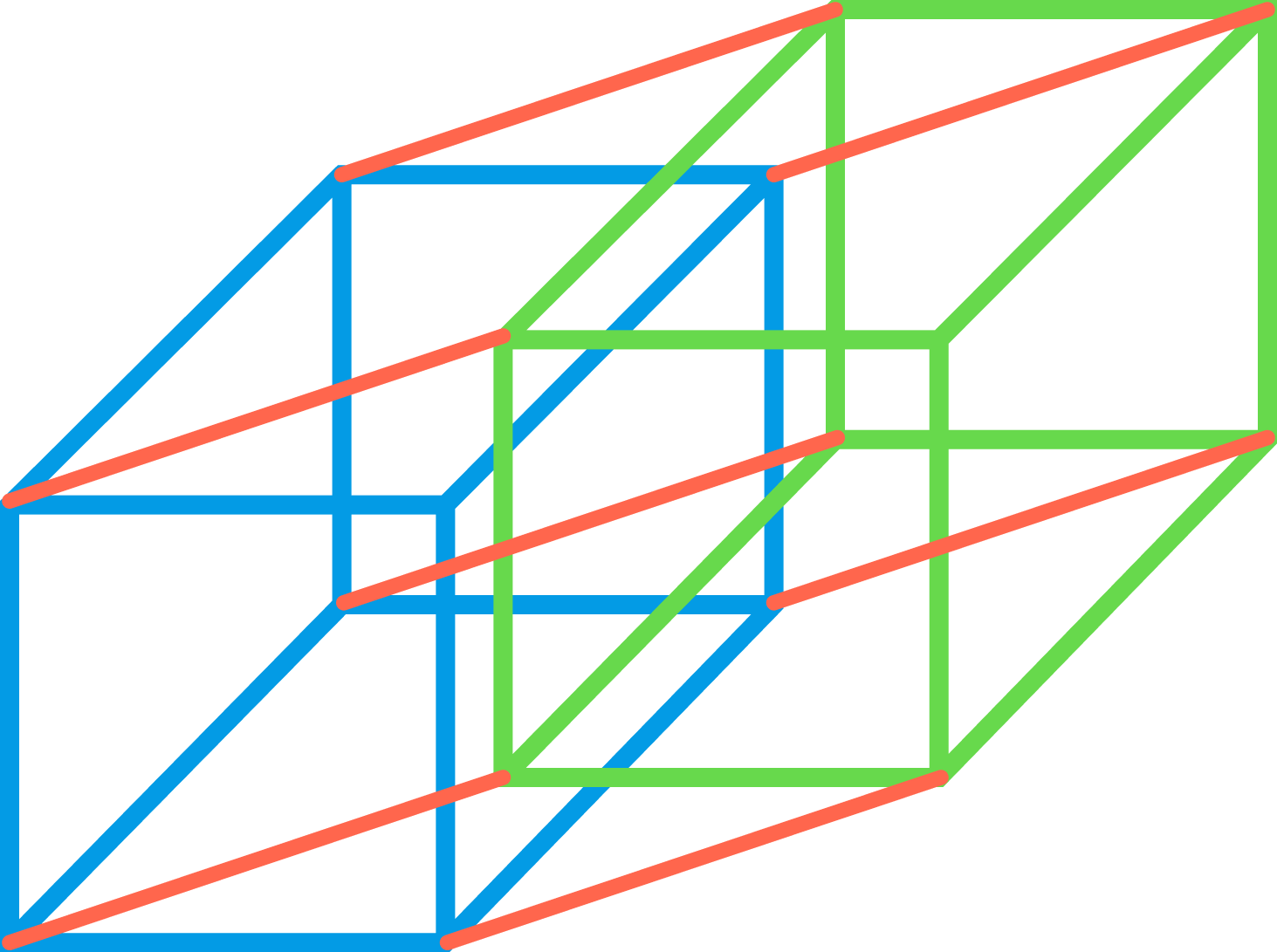# Cube GraphDefine the cube graph $C_n$ as follows:

There are $2^n$ vertices, labelled $v_0, v_1, \ldots, v_{2^n-1}$. Draw an edge between $v_a$ and $v_b$ if and only if the binary representations of $a$ and $b$ differ in exactly one digit.

We would like to find a path along the cube graph $C_n$ that crosses each edge exactly once, and begins and ends at the same vertex. For $C_2$ this is possible: start at 0, move to 2, move to 3, move to 1, move back to 0.

What about for $n = 3$ or $n = 4 ?$

×